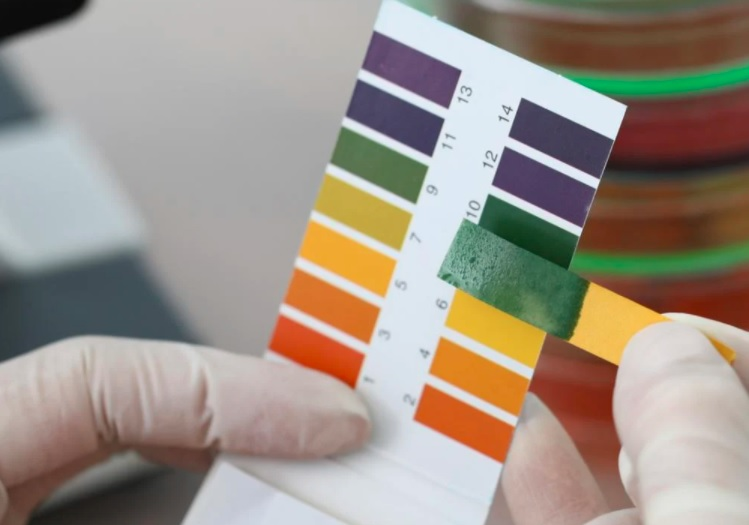# 8 Real-Life Applications for Math Equations You Learned in High School

Math equations impact the world in many interesting ways. Read on to learn about eight common applications for high school math in real world.Amanda Push
Author

Let's face it: Math can be a polarizing subject, especially among high school students who don't think they'll ever use it again after graduation. Sometimes kids might dread their mathematics class because of the memorization needed to keep the rules and formulas straight or the exact calculations required--after all, just one stray decimal point can lead to lots of mistakes.

However, whether you realize it or not, mathematical equations are part of just about every area of your life, from architecture and cooking to tomorrow's weather forecast. It can even be found in online security and evaluating the lives at risk in the event of a natural disaster.

Some of the most common branches of math include trigonometry, calculus, algebra, geometry, and statistics. From these areas of study stem equations that can be used to explore and even construct the world for the betterment of mankind.

Study.com put together a list of the real-life uses for eight math equations, compiling resources from universities, national agencies, and online learning platforms.

## Pythagorean theorem for architectural designDragon Images // Shutterstock

The Pythagorean theorem is a fundamental mathematical equation named for the Greek mathematician Pythagoras who discovered it. The Pythagorean theorem works like this: The hypotenuse of a triangle--the side that is on the opposite side of the 90-degree angle--is equal to the sum of the squares of the other two sides of the triangle. The Pythagorean theorem has many practical, real-world applications and is used regularly in architectural design. You can see evidence of it in bridges, ramps, houses, and buildings. For example, houses utilize right triangles and the Pythagorean theorem to shape sloped roofs with the 90-degree angle located at the top.

## Sine and cosine functions: Calculating force on space and aircraft flightsFrame Stock Footage // Shutterstock

You probably learned about sine and cosine functions in a high school precalculus class. In their simplest terms, sine and cosine are mathematical equations that determine the size, shape, and angle of a right triangle. The functions serve an important role in both space and aircraft flights and are used by aerospace engineers at institutions like NASA. A shuttle that's sent into space may be used to orbit the Earth. To accomplish this, scientists need to calculate the shuttle's orbit curve in relation to the Earth's equator, creating an orbit curve that's similar to a sine curve.

Looking for geometry tutoring?

## Logarithms for measuring pHH_Ko // Shutterstock

Back in high school chemistry, you might recall dipping tiny strips of paper into substances and watching whether the paper turned a different color. In these instances, you were most likely testing the acidity of a substance or solution, or measuring the pH scale. To do this, you need to utilize logarithms in your equations. A logarithm is defined as what power a number must be increased to in order to reach a different value. Measuring pH is important when it comes to measuring the acidity in everyday substances such as water and soil. Chemists also need to balance the correct pH in household products including cleaners, dishwashing detergent, toothpaste, and skincare products to ensure they're safe and effective.

## Probability equations for disaster riskelRoce // Shutterstock

Probability equations are similar to how they sound--mathematical equations used to predict the likelihood of an event. Probability equations serve an important purpose when it comes to evaluating disaster risk. For example, the Office of Disaster Preparedness and Management calculates risk by multiplying the 'probability of hazard' by the degree of vulnerability. If a natural disaster, such as a hurricane, was expected to impact a certain region, scientists would perform a disaster risk assessment using probability equations to evaluate the potential liability and loss to property and even human life. Scientists weigh acceptable risk and residual risk which, respectively, is risk that is deemed tolerable and risk that may occur even when preventive measures are in place.

## Exponential functions for bacterial growth and decaySmartPhotoLab // Shutterstock

If you ever studied biology in high school, you may remember exponential functions being mentioned. This equation can be used to measure population growth over time. Exponential functions are often used in biological studies to measure bacterial growth and decay. For example, if a bacterial cell splits itself into two, then each of those two cells split into two, you'll quickly have bacteria cells in numbers of four, then eight, then 16, then 32, and so on. The use of exponential functions is important to science as it can help track the growth of diseases and viruses.

## Fibonacci series for cryptologyRawpixel.com // Shutterstock

The Fibonacci series is a sequence of numbers in which each consecutive number is equal to the sum of the two numbers that come before it. For instance, if you start with the number zero, this is what a Fibonacci series would look like: 0, 1, 1, 2, 3, 5, 8, 13, and so on. The Fibonacci series is famously found in nature--trees, flowers, and other naturally occurring spiral structures grow leaves and petals that follow the sequence. It's also particularly useful when it comes to cryptology, the study of codes and how to solve them. With modern technology, the Fibonacci series can be used to encrypt sensitive information over the internet for security purposes.

## Fractions for cooking